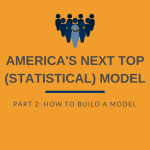## Rootscamp’s Next Top Model: How to Build a Model# Rootscamp’s Next Top Model: How to Build a Model

Quick Tip

This post is the second part of a three-part series based on a training given at NOI’s RootsCamp Conference in December.  Read part one to learn what statistical models are and check out part three to figure out how accurate a model is.

Sampling

Now that we know what a model is, we’re going to talk about what makes up a model. Models are made from a sample of the data.  Generally we do not have data on everyone or everything that we are interested in, but we have data on a small sample of them.  Often this data comes in the form of a survey.  For example, we might know who our survey respondents will vote for; we can use this information to model voting preferences for the rest of the voter file.

The chart  below represents everyone in a particular district.  Each dot represents one person.  We know their ages, and whether they will vote for for the Republican (the red dots) or the Democrat (the blue dots).  The black line is a model that shows the probability that a randomly selected voter will vote for the Republican if we know their age.  You can see that in this district, the older voters are more likely to vote Republican.Since it is usually impossible to poll everyone in a district, we often only have data on how a small group of randomly selected voters will vote.  The chart on the left below represents the ten voters in our district that we interviewed in our poll.  On the right, the black line represents a model built with our small sample, and the grey line represents the “true” model that was taken from the chart above.  The black line does a reasonable job of approximating the “true” model, but it is not perfect.  A larger poll would reduce the error in this approximation (bringing the black line closer to the grey) in the same way that larger polls have smaller margins of error.

Transformations

Once we have data from our sample, there are several decisions that must be made when building the model.  Many of these decisions are very subjective–this is where model building becomes more of an art than a science.  Two of these decisions that we must make are how to transform variables and what type of model to use.

Often, a model can make better predictions when some of the variables are transformed.  Transforming variables is just a fancy term for plugging the numbers into an equation.  For example we could transform someone’s age (let’s say they are 30 years old), with a “square” transformation.  The new age-squared variable would now equal 900 (30 squared) for this person.

In our voting example, a variable transformation would improve the model because the relationship between age and voting is not as direct as the relationship between age-squared and voting.  There are many other ways to mathematically transform a variable like age (1/age, square-root(age), etc.).  The math here can get a little complicated, but the main idea is that there are many ways of transforming variables but no clear rules about which transformations to use.  It takes some practice and a lot of subjective judgement to determine how to transform variables for a model.

Choosing a Type of Model

In the previous post, we discussed linear and logistic regression.  These are two very popular types of models even though they were invented almost 200 years ago.  In fact, these guys could have used logistic regression to model voters when they were running for president:

Linear and logistic regression were created to be calculated by hand–without the aid of a computer.  Recently, many new modeling techniques have been invented that take advantage of computers to manipulate data in ways that would have been impossible back in the days of Van Buren.  These new techniques (with funny names like support vector machines, random forest, and bagging) use different algorithms to make predictions.  We won’t go into these techniques here, but if you want to learn more about them, you can take a great online class.

Each algorithm uses slightly different assumptions about the data to make slightly different predictions.  Which algorithm is the most accurate depends on the structure of your data.  It is usually quite difficult to know which algorithm will work best in a given situation, we often rely on experience and subjective judgement in deciding which algorithm to use.

That’s it for now!  In the final part of this series, we will discuss how to evaluate the quality of a model.

## Written By